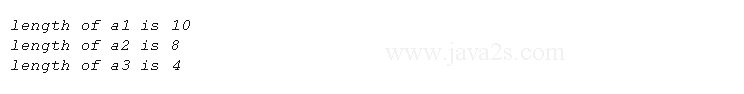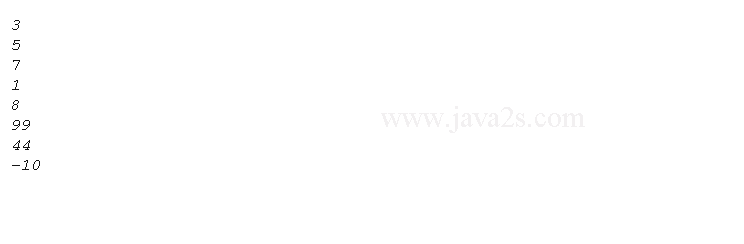# Java Array length property

## Introduction

Java arrays are implemented as objects.

The size of an array is the number of elements that an array is holding.

We can access array length via its length instance variable.

Here is a program that demonstrates this property:

```// demonstrates the length array member.
public class Main {
public static void main(String args[]) {
int a1[] = new int;
int a2[] = {3, 5, 7, 1, 8, 99, 44, -10};
int a3[] = {4, 3, 2, 1};

System.out.println("length of a1 is " + a1.length);
System.out.println("length of a2 is " + a2.length);
System.out.println("length of a3 is " + a3.length);
}//  ww  w.  ja  v  a  2 s. c om
}
```The following code uses `length` properties in a for loop.

```public class Main {
public static void main(String args[]) {
int a[] = {3, 5, 7, 1, 8, 99, 44, -10};
for(int i=0;i<a.length;i++) {
System.out.println(a[i]);//from   www .  jav  a  2  s  .  c  om
}

}
}
```